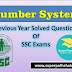## Number System: Quantitative Aptitude Questions SET-1

In this post we are sharing previous year questions of Number System that are asked in various SSC Exam like SSC CGL, SSC CHSL, SSC CPO, SSC DELHI SI, SSC SI and many other. Practice these set of questions that are very useful for your next exam.

1. Which of the following fraction is the smallest?
$\frac{7}{6}$, $\frac{7}{9}$, $\frac{4}{5}$, $\frac{5}{7}$

(1) $\frac{7}{6}$ (2) $\frac{7}{9}$
(3) $\frac{4}{5}$ (4) $\frac{5}{7}$
SSC CGL Exam. 04.07.1999 (Ist Sitting)
2. Which of the following fraction is the smallest?
$\frac{9}{13}$, $\frac{17}{26}$, $\frac{28}{29}$, $\frac{33}{52}$

(1) $\frac{33}{52}$ (2) $\frac{17}{26}$
(3) $\frac{9}{13}$ (4) $\frac{28}{29}$
SSC CGL Exam. 04.07.1999 (IIst Sitting)
3. The smallest possible threeplace decimal number is :

(1) 0.012 (2) 0.123
(3) 0.111 (4) None of the above
SSC CGL Exam. 27.02.2000 (IInd Sitting)
4. Which of the following fraction is the smallest?
$\frac{8}{15}$, $\frac{14}{33}$, $\frac{7}{13}$, $\frac{11}{13}$

(1) $\frac{8}{15}$ (2) $\frac{7}{13}$
(3) $\frac{11}{13}$ (4) $\frac{14}{33}$
SSC CGL Exam. 24.02.2002 (Ist Sitting)
5. Which of the following fraction is the smallest?
$\frac{8}{25}$, $\frac{7}{23}$, $\frac{11}{23}$, $\frac{14}{53}$

(1) $\frac{8}{25}$ (2) $\frac{7}{23}$
(3) $\frac{11}{23}$ (4) $\frac{14}{53}$
SSC CGL Prelim Exam. 24.02.2002 (Middle Zone)

6. Which of the following is the largest fraction?
$\frac{6}{7}$, $\frac{5}{6}$, $\frac{7}{8}$, $\frac{4}{5}$

(1) $\frac{6}{7}$ (2) $\frac{4}{5}$
(3) $\frac{5}{6}$ (4) $\frac{7}{8}$
SSC CGL Prelim Exam. 11.05.2003 (First Sitting)
7. The smallest number of five digits exactly divisible by 476 is:

(1) 47600 (2) 10000
(3) 10476 (4) 10472
SSSC CGL Prelim Exam. 08.02.2004 (First Sitting)
8. The least among the fractions is:
$\frac{15}{16}$, $\frac{19}{20}$, $\frac{24}{25}$, $\frac{34}{35}$

(1) $\frac{34}{35}$ (2) $\frac{15}{16}$
(3) $\frac{19}{20}$ (4) $\frac{24}{25}$
SSC CGL Tier-I Exam. 16.05.2010 (Second Sitting)
9. The greatest fraction among $\frac{2}{3}$, $\frac{5}{6}$, $\frac{11}{15}$ and $\frac{7}{8}$ is:

(1) $\frac{7}{8}$ (2) $\frac{11}{15}$
(3) $\frac{5}{6}$ (4) $\frac{2}{3}$
SSC CISF ASI Exam. 29.08.2010 (Paper-1)
10. The least number among $\frac{4}{9}$, $\sqrt{\frac{9}{49}}$, 0.45 and $(0.8)^2$ is:

(1) $\frac{4}{9}$ (2) $\frac{9}{49}$
(3) $0.45$ (4) $(0.8)^2$
SSC CPO S.I. Exam. 06.09.2009
11. Which of the following number is the greatest of all??
$0.9, 0.\overline9, 0.0\overline9, 0.\overline{09}$

(1) $0.9$ (2) $0.\overline9$
(3) $0.0\overline9$ (4) $0.\overline{09}$
SSC CHSL DEO & LDC Exam. 28.11.2010 (Ist Sitting)

12. The greatest value among the fractions $\frac{2}{7}$, $\frac{1}{3}$, $\frac{5}{6}$, $\frac{3}{4}$ is:

(1) $\frac{3}{4}$ (2) $\frac{5}{6}$
(3) $\frac{1}{3}$ (4) $\frac{2}{7}$
SSC CHSL DEO & LDC Exam. 21.10.2012 (IInd Sitting)
13. The least number of five digits which has 123 as a factor is

(1) 10037(2) 10086
(3) 10081(4) 10063
SSC Delhi Police SI Exam. 19.08.2012)
14. The largest among the numbers ${(0.1)}^2$, $\sqrt{0.0121}$, $0.12$ and $\sqrt{0.0004}$ is

(1) ${(0.1)}^2$
(2) $\sqrt{0.0121}$
(3) $0.12$
(4) $\sqrt{0.0004}$
SSC CHSL DEO & LDC Exam. 28.10.2012, Ist Sitting
15. The greatest among the following numbers ${(3)}^{\frac{1}{3}}, {(2)}^{\frac{1}{2}}, 1, {(6)}^{\frac{1}{6}}$

(1) ${(2)}^{\frac{1}{2}}$ (2) $1$
(3) ${(6)}^{\frac{1}{6}}$ (4) ${(3)}^{\frac{1}{3}}$
SSC CAPFs SI & CISF ASI Exam. 23.06.2013)
16. When 335 is added to 5A7, the result is 8B2. 8B2 is divisible by 3. What is the largest possible value of A?

(1) 8 (2) 2
(3) 1 (4) 4
SSC CGL Tier-II Exam. 29.09.2013)

17. If a number is as much greater than 31 as it is less than 75, then the number is
(1) 106(2) 44
(3) 74 (4) 53
SSC CHSL DEO & LDC Exam. 20.10.2013)
18. The greatest number among $0.7+\sqrt{0.16}$, $1.02-\frac{0.6}{24}$, $1.2×0.83$ and $\sqrt{1.44}$ is:

(1) $0.7+\sqrt{0.16}$
(2) $\sqrt{1.44}$
(3) $1.2×0.83$
(4) $1.02-\frac{0.6}{24}$
SSSC CGL Prelim Exam. 08.02.2004 (Second Sitting)
19. Which is the largest of the following fractions?
$\frac{2}{3}$, $\frac{3}{5}$, $\frac{8}{11}$, $\frac{11}{17}$

(1) $\frac{8}{11}$ (2) $\frac{3}{5}$
(3) $\frac{11}{17}$ (4) $\frac{2}{3}$
SSC CGL Tier-I Re-Exam. (2013) 27.04.2014
20. Sum of three fractions is $2\frac{11}{24}$. On dividing the largest fraction by the smallest fraction, $\frac{7}{6}$ is obtained which is $\frac{1}{3}$ greater than the middle fraction. The smallest fraction is

(1) $\frac{5}{8}$ (2) $\frac{3}{4}$
(3) $\frac{5}{6}$ (4) $\frac{3}{7}$
SSC CGL Tier-II Exam, 2014 12.04.2015 (Kolkata Region)
21. Arrangement of the fractions $\frac{4}{3}$, $-\frac{2}{9}$, $-\frac{7}{8}$, $\frac{5}{12}$ into ascending order is

(1) $-\frac{7}{8}$, $-\frac{2}{9}$, $\frac{5}{12}$, $\frac{4}{3}$
(2) $-\frac{7}{8}$, $-\frac{2}{9}$, $\frac{4}{3}$, $\frac{5}{12}$
(3) $-\frac{2}{9}$, $-\frac{7}{8}$, $\frac{5}{12}$, $\frac{4}{3}$
(4) $-\frac{2}{9}$, $-\frac{7}{8}$, $\frac{4}{3}$, $\frac{5}{12}$
SSC CHSL (10+2) LDC, DEO & PA/SA Exam, 20.12.2015
22. Which of the following is correct?
(1) $\frac{2}{3}<\frac{3}{5}<\frac{11}{15}$
(2) $\frac{3}{5}<\frac{2}{3}<\frac{11}{15}$
(3) $\frac{11}{15}<\frac{2}{3}<\frac{3}{5}$
(4) $\frac{3}{5}<\frac{11}{15}<\frac{2}{3}$
SSC CGL Tier-II Online Exam.01.12.2016)

Number System: Quantitative Aptitude Questions of Previous SSC Exams With Solution SET-1Reviewed by Super Pathshala on 07:56 Rating: 5